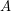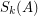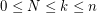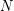# Elementary symmetric of a sum of matrices

 Importance: High ✭✭✭
 Author(s):
 Subject: Algebra
 Keywords:
 Posted by: rscosa on: December 8th, 2008
Problem

Given a Matrix, the-th elementary symmetric function of, namely, is defined as the sum of all-by-principal minors.

Find a closed expression for the-th elementary symmetric function of a sum of N-by-matrices, withby using partitions.

The Newton-Girard formulas imply particular expressions for small values ofand, for example,.

## Bibliography

* indicates original appearance(s) of problem.

that's ok